# THE BEST FORMULA FOR SOLVING QUESTIONS ON FARADAY'S LAWS OF ELECTROLYSIS

in #science3 years ago (edited)

Hello guys, I got an inspiration on this formula 8 years ago while I was preparing for JAMB exam. This formula has been helpful in solving questions on Faraday's Second Law of Electrolysis over the years. I really want you guys to see this, though some of us know what electrolysis is all about but for the sake of others reading this post, I will start by introducing electrolysis.

*In Chemistry, electrolysis is a technique or process that uses a direct electric current (DC) to drive a non- spontaneous chemical reaction. Electrolysis is commercially important as a stage in the separation of elements from naturally occurring sources such as ores using an electrolytic cell.

*An electrolytic cell consists of 2 electrodes in a molten salt or electrolyte solution. A battery or other voltage source is attached across the electrodes and serves as"electrode pump"drawing electrons from what would have been the positive electrode and forcing them in at what would have been negative.

NOTE: Reduction half-reaction occurs at the electrode (cathode) attached to the negative terminal of the battery and oxidation half-reaction occurs at the electrode (anode) attached to the positive terminal of the battery.

* LAWS OF ELECTROLYSIS

The quantitative aspect of electrolysis is summarized in the two empirical statements known as "Faraday's Laws of Electrolysis".

First law of electrolysis states that the mass (m) of a substance liberated or deposited at an electrode during an electrolysis is directly proportional to the quantity of electricity (Q) passed through the electrolyte.

![IMG_20180409_034924.jpg](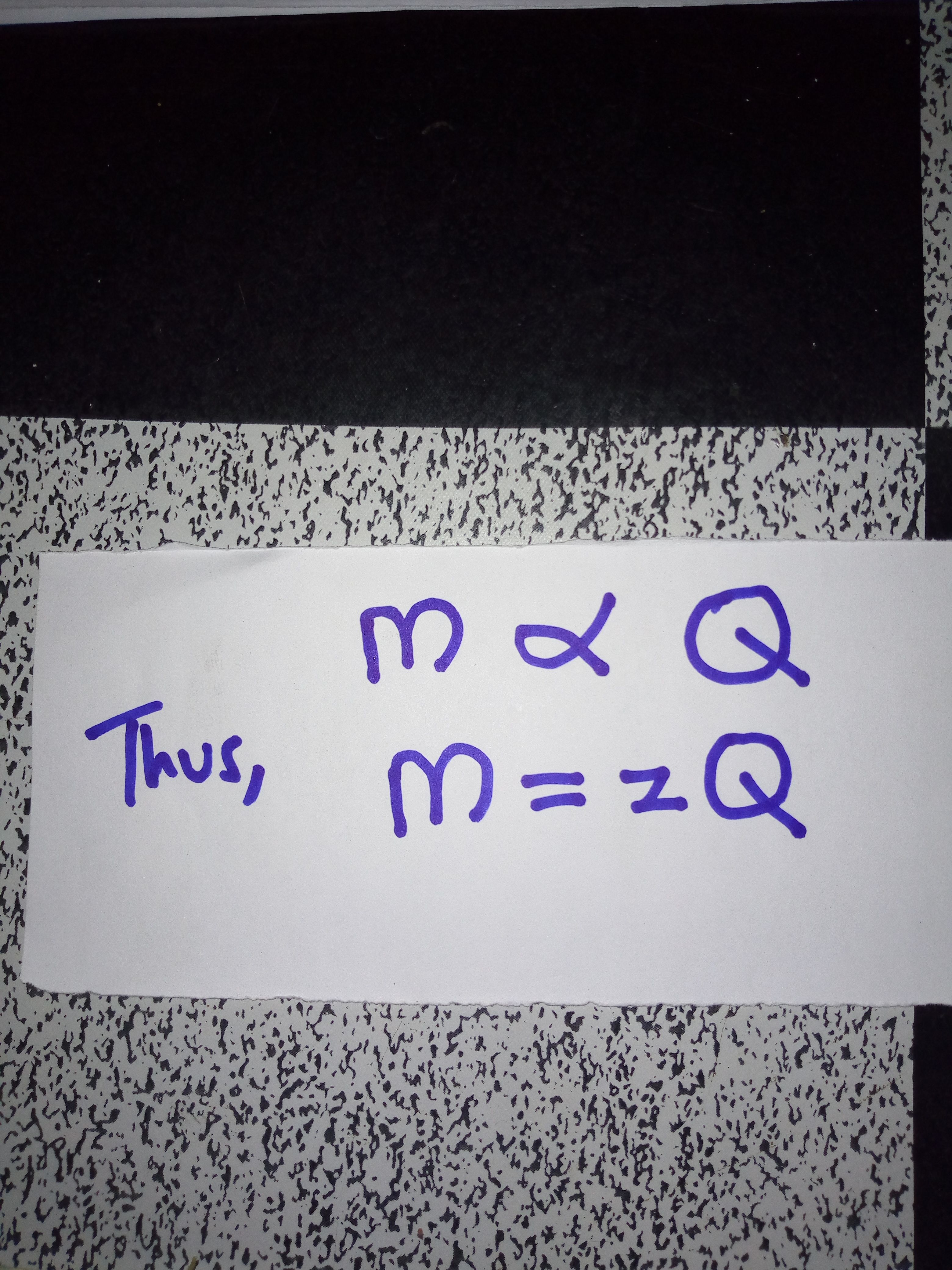)

where, z is the electrochemical equivalent of the substance.

The quantity of electricity (Q)(incoulombs) is the product of the current (I) (measured in amperes) and the time (t)(measured in seconds). That is:

![IMG_20180409_035613.jpg]()

Second law of electrolysis states that when the same quantity of electricity is passed through solutions of different electrolytes arranged in series, the relative number of moles of the elements deposited or liberated are Inversely proportional to the charges on the ions of each of elements respectively.

![IMG_20180409_040140.jpg](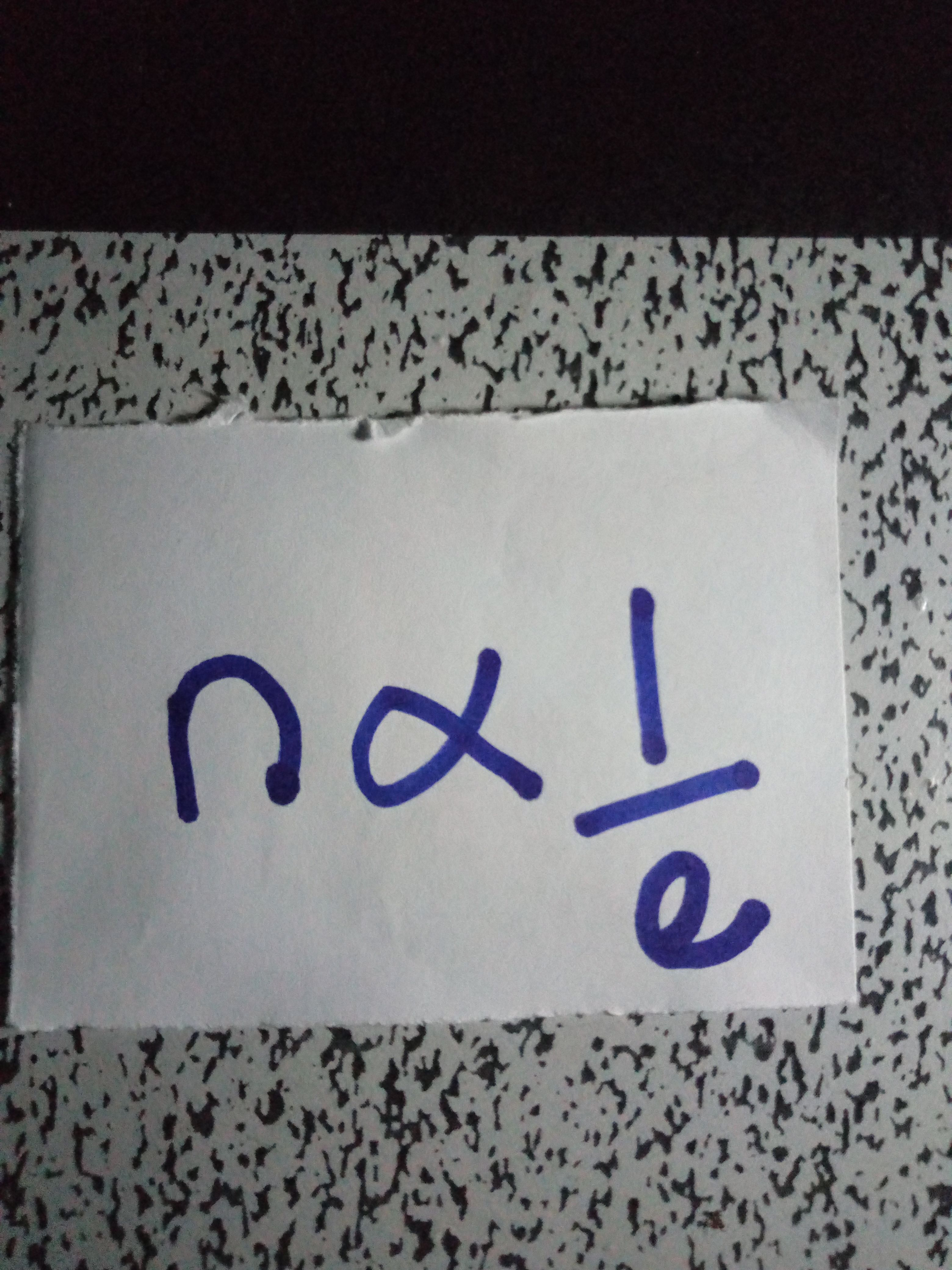)

* Here's my formula for solving questions on Faraday's Second Law of Electrolysis.

![IMG_20180409_040727_042.jpg]()

where,

n is the number of moles of electrons.

Q is the quantity of electricity

* The formula above can be re-written as;

![IMG_20180409_041142_509.jpg](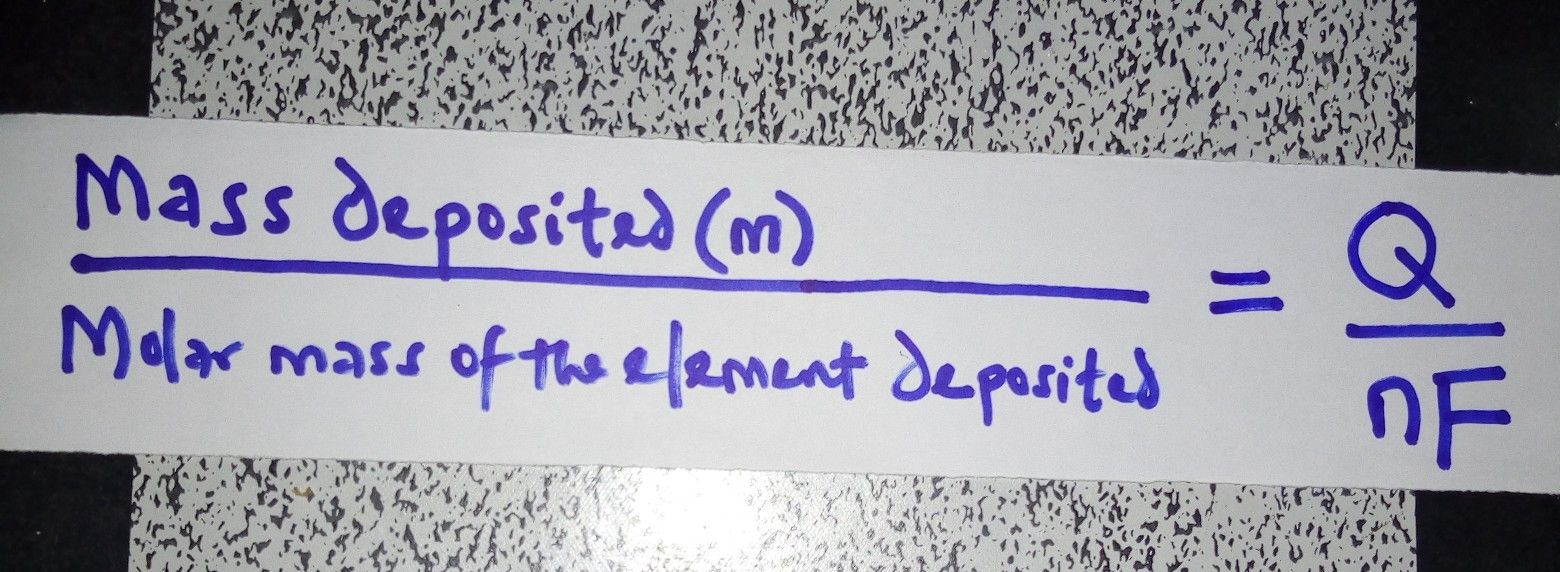)

![IMG_20180409_041425_281.jpg]()

![IMG_20180409_041809_375.jpg](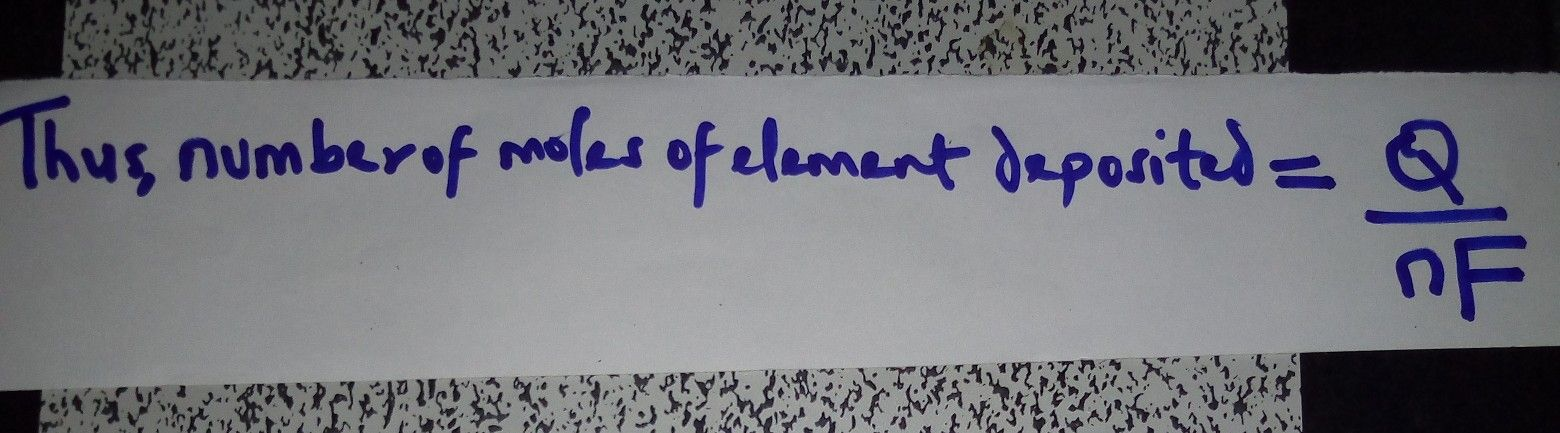)

![IMG_20180409_045244.jpg]()

![IMG_20180409_045511.jpg](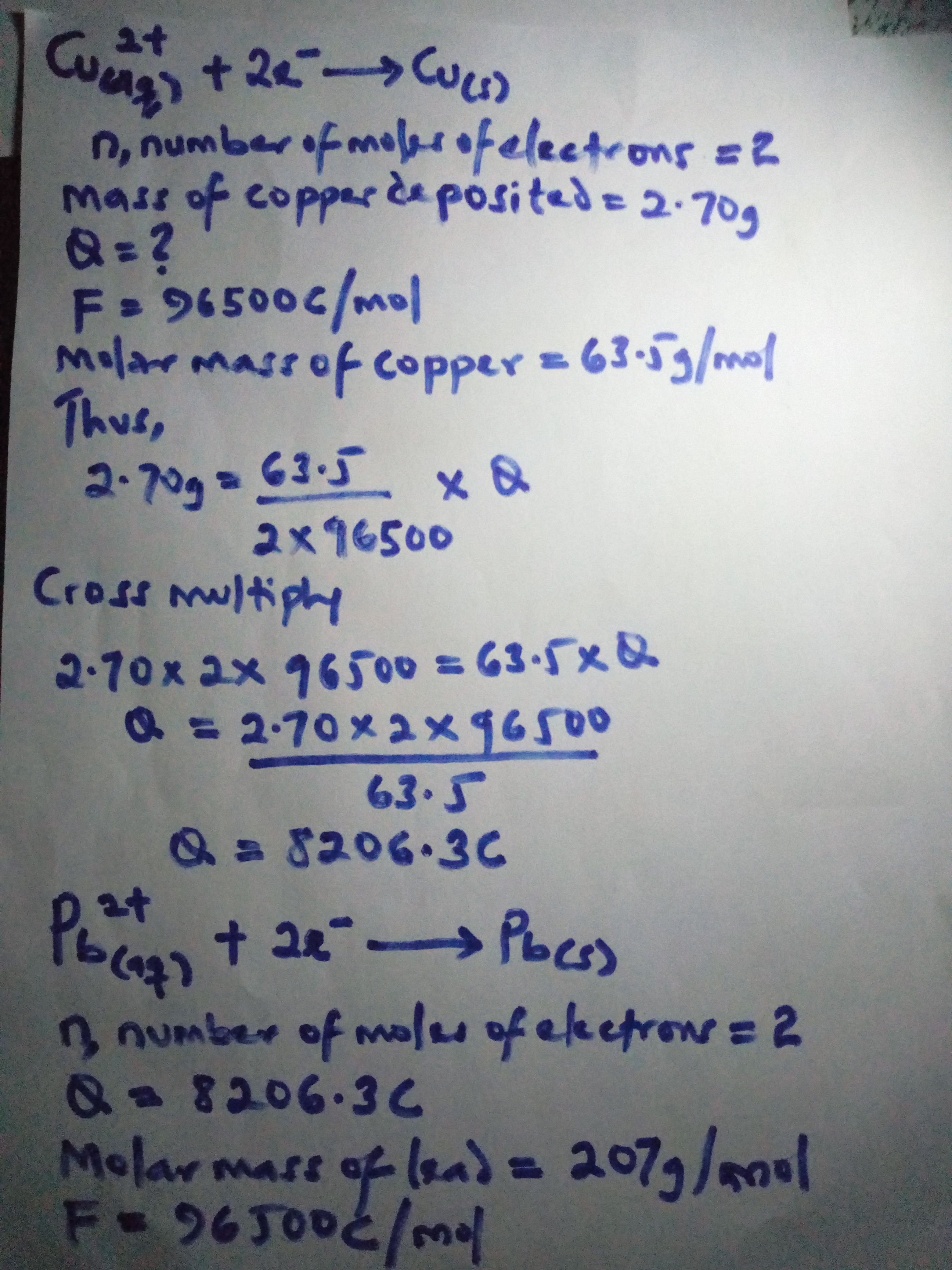)

![IMG_20180409_045822.jpg]()

Reference text: Lamlad's SSCE and JME chemistry by F. O. Ayinde and F. O. I. Asubiojo.

Kindly upvote, comment, resteem and follow.

God bless....

Sort:

Rewriting equations and formulas in your own words is the best way to internalize and understand what you're doing more intuitively, which is what it seems like you are doing here.

Yes...that's correct

This is comprehensive enough

Thanks bro

Nice post bro..... tested and proved, great work keep it up

Cu(aq)2+ + 2e- -› Cu(s)

3 years ago (edited)image source